Next: Klein-Gordon Equation in Schrödinger Up: Interaction with the Electromagnetic Previous: Electromagnetic Coupling

## Charge Conjugation

Consider a positive-energy solution with charge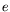. The equivalent Klein-Gordon equation satisfied by the positive-energy solution is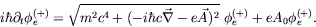(4.77)

The negative energy solution satisfies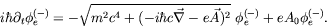(4.78)

Taking the complex conjugate of the negative energy equation gives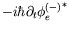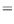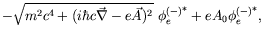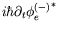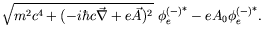(4.79)

Comparing equation 4.77 with equation 4.79 shows(4.80)

Thus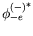is the charge conjugate solution and represents the charge conjugate state of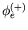. Similarly,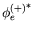is the charge conjugate state of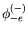. If we (arbitrarily) call the particle described bythe particle'', then we call the particle described bythe antiparticle. For example, if we call the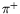meson the particle, then the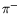meson is the antiparticle. The undesirable negative-energy solutions have now been interpreted as antiparticles.

Neutral particles fit into this picture too, in that the charge-conjugate state is the state itself. In other words, neutral particles are their own antiparticles. Let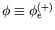and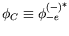so that we can write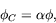(4.81)

where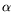is a proportionality constant which has to be real.can be deduced since for neutral particles both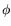and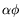are real. Realizing that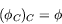(4.82)

it follows that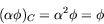(4.83)

so that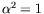and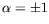. Accordingly there exist two different kinds of neutral particles, namely

1. neutral particles with positive charge parity, ie.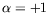,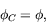2. neutral particles with negative charge parity, ie.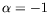,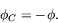Neutral particles are thus eigenfunctions of the charge conjugation operator, while charged particles are not.Next: Klein-Gordon Equation in Schrödinger Up: Interaction with the Electromagnetic Previous: Electromagnetic Coupling
Douglas M. Gingrich (gingrich@ ualberta.ca)
2004-03-18